GED Math : Area of a Quadrilateral

Example Questions

Example Question #11 : Area Of A Quadrilateral

A rectangle has length 10 inches and width 8 inches. Its length is increased by 2 inches, and its width is decreased by 2 inches. By what percent has the area of the rectangle decreased?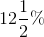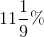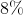Explanation:

The area of a rectangle is its length times its width.

Its original area is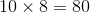square inches; its new area is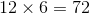square inches. The area has decreased by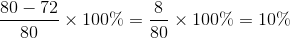.

Example Question #12 : Area Of A Quadrilateral

A rectangle has length 10 inches and width 5 inches. Each dimension is increased by 3 inches. By what percent has the area of the rectangle increased?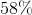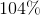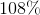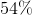Explanation:

The area of a rectangle is its length times its width.

Its original area is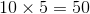square inches; its new area is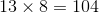square inches. The area has increased by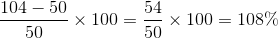.

Example Question #13 : Area Of A Quadrilateral

Find the area of a square with a side of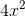.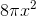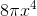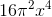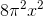Explanation:

Write the formula for the area of a square.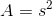Substitute the side into the equation.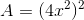Simplify the equation.

The answer is: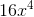Example Question #14 : Area Of A Quadrilateral

If a rectangle has a length of 18cm and a width that is half the length, what is the area of the rectangle?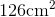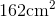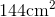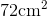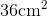Explanation:

To find the area of a rectangle, we will use the following formula: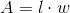where l is the length and w is the width of the rectangle.

Now, we know the length of the rectangle is 18cm. We also know the width is half the length. Therefore, the width is 9cm. So, we can substitute.  We get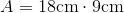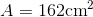Example Question #15 : Area Of A Quadrilateral

If a square has a length of 10in, find the area.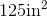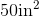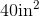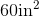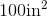Explanation:

To find the area of a square, we will use the following formula:where l is the length and w is the width of the square.

Now, we know the length of the square is 10in. Because it is a square, all sides are equal. Therefore, the length is also 10in. So, we can substitute. We get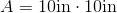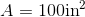Example Question #16 : Area Of A Quadrilateral

Find the area of a rectangle with a width of 8in and a length that is two times the width.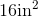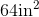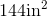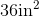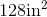Explanation:

To find the area of a rectangle, we will use the following formula:where l is the length and w is the width of the rectangle.

Now, we know the width of the rectangle is 8in. We also know the length of the rectangle is two times the width. Therefore, the length is 16in. So, we can substitute. We get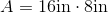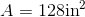Example Question #17 : Area Of A Quadrilateral

Find the area of a square with a length of 11cm.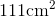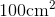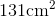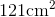Explanation:

To find the area of a square, we will use the following formula:where l is the length and w is the width of the square.

Now, we know the length of the square is 11cm. Because it is a square, all sides are equal. Therefore, the width is also 11cm.  So, we can substitute. We get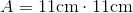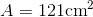Example Question #18 : Area Of A Quadrilateral

Find the area of a rectangle with a width of 7in and a length that is three times the width.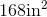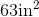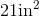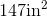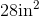Explanation:

To find the area of a rectangle, we will use the following formula:where l is the length and w is the width of the rectangle.

Now, we know the width of the rectangle is 7in. We also know the length is three times the width. Therefore, the length is 21in. So, we can substitute. We get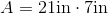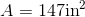Example Question #19 : Area Of A Quadrilateral

A square has an area of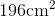.  Find the length of one side.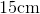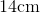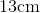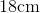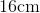Explanation:

A square has 4 equal sides. The formula to find the area of a square is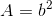where b is the length of one side of the square. To find the length of one side of the square, we will solve for b.

Now, we know the area of the square is. So, we will substitute and solve for b. So,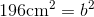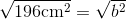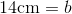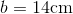Therefore, the length of one side of the square is 14cm.

Example Question #20 : Area Of A Quadrilateral

What of the following is NOT a property of a quadrilateral?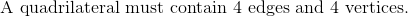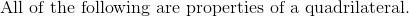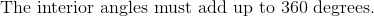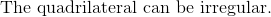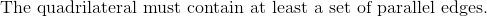Explanation:

A quadrilateral is a shape with four corners, or vertices, whose interior angles angles must sum up to 360 degrees.

The shape also has 4 edges.

The quadrilateral does not necessarily require two pairs of parallel lines.